# CS767_Lecture_02.pptx

5. Sep 2022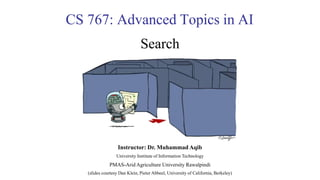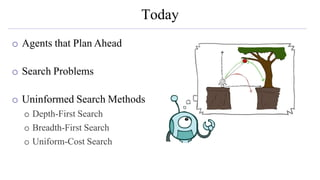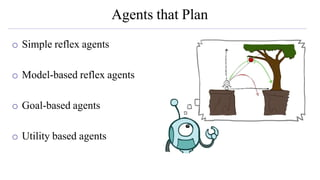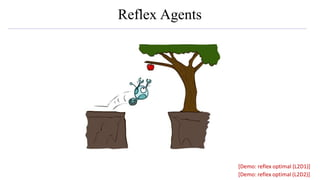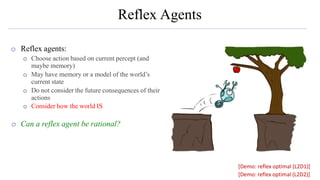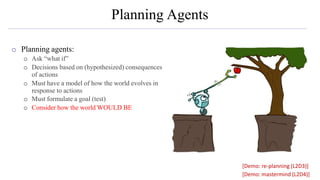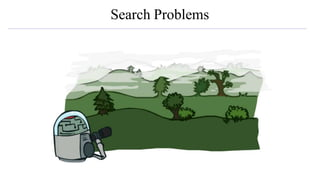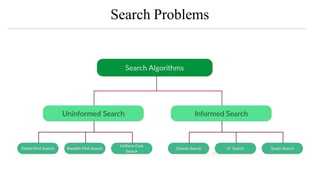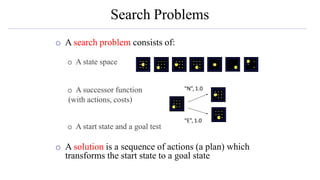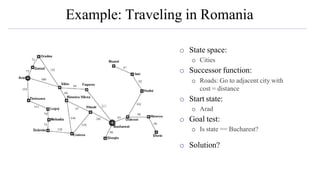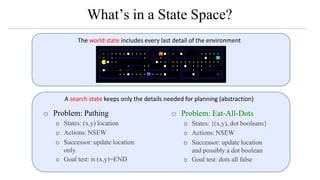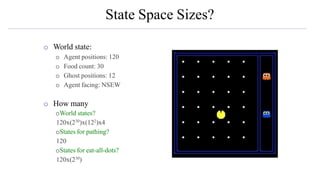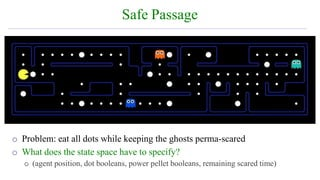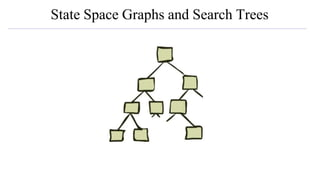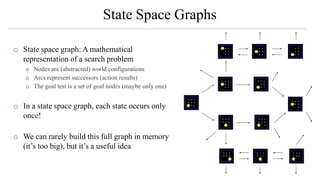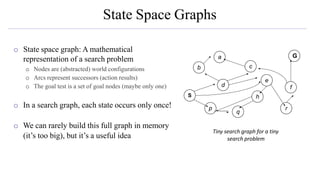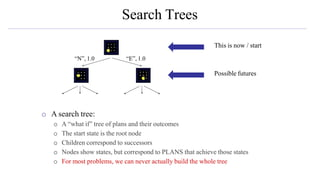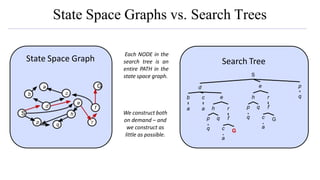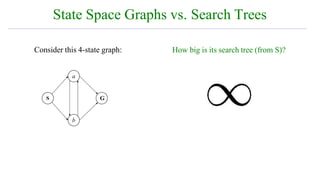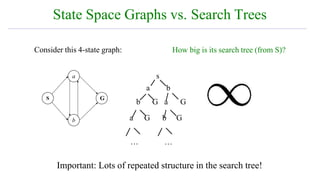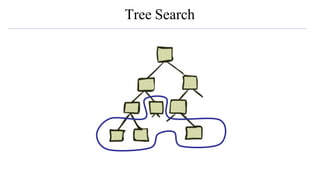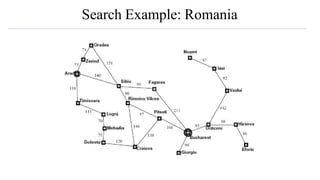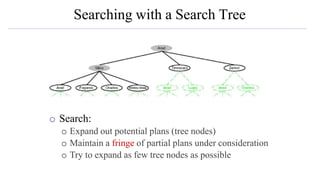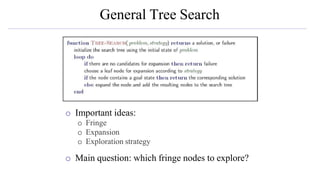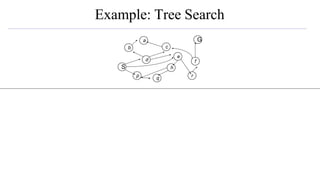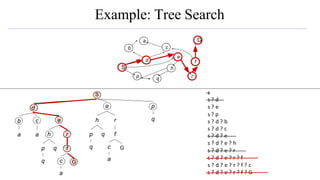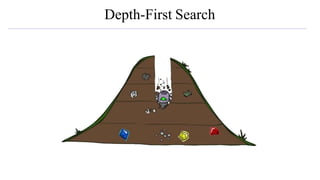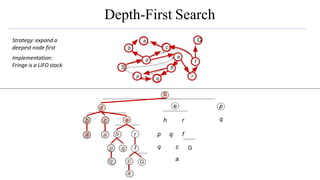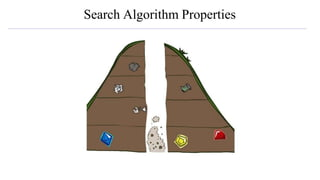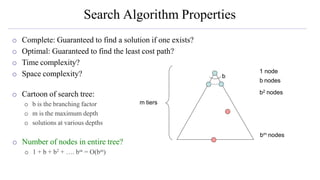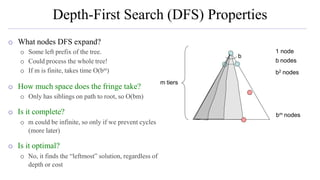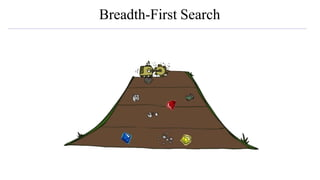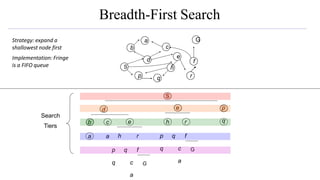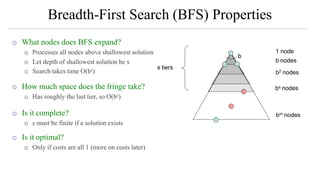1 von 35

### CS767_Lecture_02.pptx

• 1. CS 767: Advanced Topics in AI Search Instructor: Dr. Muhammad Aqib University Institute of Information Technology PMAS-Arid Agriculture University Rawalpindi (slides courtesy Dan Klein, Pieter Abbeel, University of California, Berkeley)
• 2. Today o Agents that Plan Ahead o Search Problems o Uninformed Search Methods o Depth-First Search o Breadth-First Search o Uniform-Cost Search
• 3. Agents that Plan o Simple reflex agents o Model-based reflex agents o Goal-based agents o Utility based agents
• 4. Reflex Agents [Demo: reflex optimal (L2D1)] [Demo: reflex optimal (L2D2)]
• 5. Reflex Agents o Reflex agents: o Choose action based on current percept (and maybe memory) o May have memory or a model of the world’s current state o Do not consider the future consequences of their actions o Consider how the world IS o Can a reflex agent be rational? [Demo: reflex optimal (L2D1)] [Demo: reflex optimal (L2D2)]
• 6. Planning Agents o Planning agents: o Ask “what if” o Decisions based on (hypothesized) consequences of actions o Must have a model of how the world evolves in response to actions o Must formulate a goal (test) o Consider how the world WOULD BE [Demo: re-planning (L2D3)] [Demo: mastermind (L2D4)]
• 7. Search Problems
• 8. Search Problems
• 9. Search Problems o A search problem consists of: o A state space o A successor function (with actions, costs) o A start state and a goal test o A solution is a sequence of actions (a plan) which transforms the start state to a goal state “N”, 1.0 “E”, 1.0
• 10. Example: Traveling in Romania o State space: o Cities o Successor function: o Roads: Go to adjacent city with cost = distance o Start state: o Arad o Goal test: o Is state == Bucharest? o Solution?
• 11. What’s in a State Space? o Problem: Pathing o States: (x,y) location o Actions: NSEW o Successor: update location only o Goal test: is (x,y)=END o Problem: Eat-All-Dots o States: {(x,y), dot booleans} o Actions: NSEW o Successor: update location and possibly a dot boolean o Goal test: dots all false The world state includes every last detail of the environment A search state keeps only the details needed for planning (abstraction)
• 12. State Space Sizes? o World state: o Agent positions: 120 o Food count: 30 o Ghost positions: 12 o Agent facing: NSEW o How many oWorld states? 120x(230)x(122)x4 oStates for pathing? 120 oStates for eat-all-dots? 120x(230)
• 13. Safe Passage o Problem: eat all dots while keeping the ghosts perma-scared o What does the state space have to specify? o (agent position, dot booleans, power pellet booleans, remaining scared time)
• 14. State Space Graphs and Search Trees
• 15. State Space Graphs o State space graph: A mathematical representation of a search problem o Nodes are (abstracted) world configurations o Arcs represent successors (action results) o The goal test is a set of goal nodes (maybe only one) o In a state space graph, each state occurs only once! o We can rarely build this full graph in memory (it’s too big), but it’s a useful idea
• 16. State Space Graphs o State space graph: A mathematical representation of a search problem o Nodes are (abstracted) world configurations o Arcs represent successors (action results) o The goal test is a set of goal nodes (maybe only one) o In a search graph, each state occurs only once! o We can rarely build this full graph in memory (it’s too big), but it’s a useful idea S G d b p q c e h a f r Tiny search graph for a tiny search problem
• 17. Search Trees o A search tree: o A “what if” tree of plans and their outcomes o The start state is the root node o Children correspond to successors o Nodes show states, but correspond to PLANS that achieve those states o For most problems, we can never actually build the whole tree “E”, 1.0 “N”, 1.0 This is now / start Possible futures
• 18. State Space Graphs vs. Search Trees a b d p a c e h r p q f q G c a q e h r p q f q G c a Search Tree S S G d b p q c e h a f r We construct both on demand – and we construct as little as possible. Each NODE in the search tree is an entire PATH in the state space graph. State Space Graph
• 19. State Space Graphs vs. Search Trees S G b a Consider this 4-state graph: How big is its search tree (from S)?
• 20. State Space Graphs vs. Search Trees S G b a Consider this 4-state graph: … … Important: Lots of repeated structure in the search tree! How big is its search tree (from S)? s a b b G a G a G b G
• 21. Tree Search
• 22. Search Example: Romania
• 23. Searching with a Search Tree o Search: o Expand out potential plans (tree nodes) o Maintain a fringe of partial plans under consideration o Try to expand as few tree nodes as possible
• 24. General Tree Search o Important ideas: o Fringe o Expansion o Exploration strategy o Main question: which fringe nodes to explore?
• 25. Example: Tree Search S G d b p q c e h a f r
• 26. Example: Tree Search a a p q f q h r c a G q q S G b p q c h a f d e r S d e p e h r p q f c a G b c s s ? d s ? e s ? p s ? d ? b s ? d ? c s ? d ? e s ? d ? e ? h s ? d ? e ? r s ? d ? e ? r ? f s ? d ? e ? r ? f ? c s ? d ? e ? r ? f ? G
• 27. Depth-First Search
• 28. Depth-First Search S a b d p a c e h r p q f q G c a q e h r p q f q G c a S G q p h f d b a c e r Strategy: expand a deepest node first Implementation: Fringe is a LIFO stack
• 29. Search Algorithm Properties
• 30. Search Algorithm Properties o Complete: Guaranteed to find a solution if one exists? o Optimal: Guaranteed to find the least cost path? o Time complexity? o Space complexity? o Cartoon of search tree: o b is the branching factor o m is the maximum depth o solutions at various depths o Number of nodes in entire tree? o 1 + b + b2 + …. bm = O(bm) … b 1 node b nodes b2 nodes bm nodes m tiers
• 31. Depth-First Search (DFS) Properties o What nodes DFS expand? o Some left prefix of the tree. o Could process the whole tree! o If m is finite, takes time O(bm) o How much space does the fringe take? o Only has siblings on path to root, so O(bm) o Is it complete? o m could be infinite, so only if we prevent cycles (more later) o Is it optimal? o No, it finds the “leftmost” solution, regardless of depth or cost … b 1 node b nodes b2 nodes bm nodes m tiers